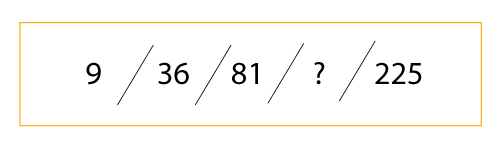⚡ Save 20% on Premium with code SAVE20

# Criteria Cognitive Aptitude Test Preparation (CCAT)Ben Hopgood Updated:

Follow this guide to better understand the CCAT test, and try our free and premium tests.

###### Page contents:

Complete the sequence below. Find what number replaces the "?" symbol. You have 20 seconds starting NOW!Have you managed to determine the correct answer?

###### Solution:

Now that you have attempted the question, you will have an answer. Let us now determine the solution.

We can notice that 9 is the square of 3. Likewise, 36 is the square of 6. The sequence is a series of square numbers. 81 = 9, and 225 = 15. We can then notice that these squares are multiples of 3. So, we have: 3, 6, ?, 15. Therefore, we require the square of 12.

Calculation: 12 x 12 = 144

Perhaps, now you're ready to have a go at a full free practice CCAT test. Have a look at the one we have below. Good luck!

###### Criteria Cognitive Aptitude Test
CCAT

This free CCAT has 16 questions and a time limit of 8 minutes. We rate this one as hard difficulty, but very typical of the CCAT.

## How do CCAT tests work?

The Criteria Cognitive Aptitude Test (CCAT) measures the candidate's ability to solve problems and think critically. It's one of the most popular cognitive assessment tests there are, and is particularly popular in the US. It has 50 questions and takes 15 minutes to complete. Therefore, the questions are quickly answered and many candidates finish all the questions before the time limit. This is a pre-employment test and is commonly given to candidates applying for a vast range of positions.

## CCAT test outline

The important thing to remember about CCAT tests used for employment selection is that they contain many different question styles. They usually do not permit calculators, so the mathematical calculations you must work out should not be too complex. The typical questions styles include but are not limited to:

• Numerical reasoning questions with graphs or charts
• Logical reasoning questions via abstract symbols
• Verbal reasoning questions and text dissemination
• Number sequences
• Deductive reasoning questions
• Synonyms and antonyms

Given the complexity of the various question types, it is important that you practice CCAT tests and get familiar with the different types of questions. Remember, they contain numerical, logical, verbal and deductive type questions, among others, and so there are a lot of skills that need refining.

The good news, we have developed a free CCAT test that you can try. Just scroll back up a little!

## 87% of users aced their employment test in 2022*

Get a completely free starter account with over 20 tests included.

*According to our 2022 survey of 1486 users